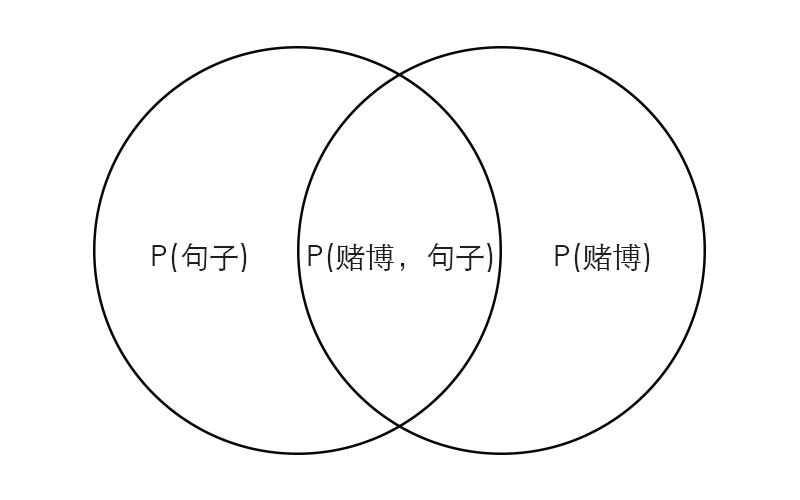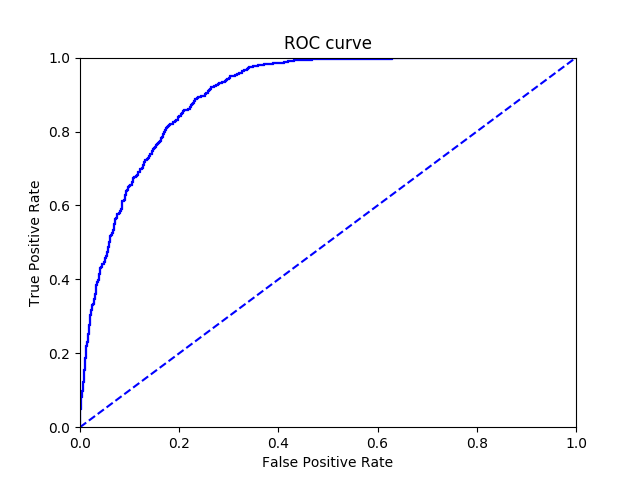# 理解贝叶斯分类

### cherry分类器

##### 基础术语：

``````>>> import cherry
>>> result = cherry.classify('警方召开了全省集中打击赌博违法犯罪活动专项行动电 电话会议。会议的重点是“查处”六合彩、赌球赌马等赌博活动。')
Building prefix dict from the default dictionary ...
Prefix dict has been built succesfully.
``````

``````>>> result.percentage
[('normal.dat', 0.997), ('politics.dat', 0.002), ('gamble.dat', 0.0), ('sex.dat', 0.0)]
``````

``````>>> result.word_list
[('赌博', 8.5881312727226), ('活动', 6.401543938544878), ('会议', 6.091963362021649), ('违法犯罪', 4.234845736802978), ('警方', 3.536827626008435), ('打击', 3.2491455535566542), ('行动', 2.8561029654470476), ('查处', 2.3860993362013083), ('重点', 2.126816738271229), ('召开', 1.8628511924367634), ('专项', 1.1697040118768172), ('电视电话会议', 1.1697040118768172), ('全省', 0.47655683131687354), ('集中', -0.6220554573512382), ('六合彩', -2.29603189092291)]
``````

### 贝叶斯模型

``````警方，召开，全省，集中打击，... 六合彩，赌球，赌马，...
``````

### 数学推导

P(A) -> A事件发生的概率，例如明天天晴的概率

P(A|B) -> 条件概率，B事件发生的前提下A事件发生的概率，例如明天天晴而我又没带伞的概率

P(输入句子) -> 这个句子在训练数据中出现的概率

P(赌博) -> 赌博类别的句子在训练数据中出现的概率

P(赌博|输入句子) -> 输入句子是赌博类别的概率（也是我们最终要求的值）

P(赌博|输入句子) + P(正常|输入句子) = 100%P(赌博|输入句子) = P(赌博，输入句子) / P(输入句子) (1)

P(输入句子|赌博) = P(赌博，输入句子) / P(赌博) (2)

P(赌博|输入句子) = P(输入句子|赌博) * P(赌博) / P(输入句子) (3)

1. P(赌博)

指训练数据中，赌博类别的句子占训练数据的百分比。

2. P(输入句子)

指这个输入句子出现在训练数据中的概率。我们最终目的是判断输入句子是哪个类别的概率比较高，也就是比较P(赌博|输入句子)与P(正常|输入句子)，由贝叶斯定理：

P(赌博|输入句子) = P(输入句子|赌博) * P(赌博) / P(输入句子) (4)

P(正常|输入句子) = P(输入句子|正常) * P(正常) / P(输入句子) (5)

由于(4)，(5)都要除于相同的P(输入句子)，所以(4)，(5)右边可以同时乘以P(句子)，只比较等号右边前两个值的乘积的大小。

P(赌博|输入句子) = P(输入句子|赌博) * P(赌博) P(正常|输入句子) = P(输入句子|正常) * P(正常)

3. P(句子|赌博)

最关键的就是求P(输入句子|赌博)，直接求输入句子在赌博类别句子中出现的概率非常困难，因为训练数据不可能包含所有句子，很可能并没有输入句子。什么意思呢？因为同一个句子，把词语进行不同的排列组合都能成立，例如：

奖金将在您完成首存后即可存入您的账户。真人荷官，六合彩，赌球欢迎来到全新番摊游戏！

可以变成

奖金将在您完成首存后即可存入您的账户。六合彩，赌球，真人荷官欢迎来到全新番摊游戏！

或者

欢迎来到全新番摊游戏，奖金将在您完成首存后即可存入您的账户。六合彩，真人荷官，赌球！

稍微变换词语的位置就是一个新的句子了，训练数据不可能把所有排列组合的句子都加进去，因为实在太多了。所以当我们遇到一个输入句子，很可能它在训练数据中没有出现，那么P(输入句子|类别)对应的概率都为零，这显然不是真实的结果。也会导致我们的分类器出错，这个时候该怎么办呢？刚刚在贝叶斯模型中我们提到，它会将一个句子分成不同的词语来综合分析，那我们是不是也可以把句子当成词语的集合呢？

警方召开了全省集中打击赌博违法犯罪活动专项行动电视电话会议。会议的重点是“查处”六合彩、赌球赌马等赌博活动。

警方召开了全省…赌马等赌博活动 = 警方 + 召开 + 全省…+赌博活动

即：

P(输入句子|赌博) = (P(词语1) * P(词语2|词语1) * P(词语3|词语2))|赌博) ≈ P(词语1)|P(赌博) * P(词语2)|P(赌博) * P(词语3)|P(赌博)

P(警方召开了全省…赌马等赌博活动。|赌博) = P(警方|赌博) * P(召开|赌博) * P(全省|赌博) … * P(赌马|赌博) * P(赌博活动|赌博)

我们把P(输入句子|赌博)分解成所有P(词语|赌博)概率的乘积，然后通过训练数据，计算每个词语在不同类别出现的概率。最终获取的是输入句子有效词语在不同类别中的概率

词语 正常 赌博
警方 0.8 0.2
召开 0.7 0.3
全省 0.7 0.3
赌马 0.4 0.6
赌球 0.3 0.7
赌博活动 0.4 0.6
——- ——- ——-
综合概率 0.8 0.2

### 贝叶斯模型实现

1. 选择训练数据，标记类别
2. 把所有训练数据进行分词，并且组成成一个包含所有词语的词袋集合
3. 把每个训练数据转换成词袋集合长度的向量
4. 利用每个类别的下训练数据，计算词袋集合中每个词语的概率

#### 选择训练数据

• 赌博类别

根据您所选择的上述六合彩游戏，您必须在娱乐场完成总金额（存款+首存奖金）16倍或15倍流水之后，方可申请提款。

奖金将在您完成首存后即可存入您的账户。真人荷官 六合彩 欢迎来到全新番摊游戏！

• 正常类别

Linux是一套免费使用和自由传播的类Unix操作系统，是一个基于POSIX和UNIX的多用户、多任务、支持多线程和多CPU的操作系统。

理查德·菲利普斯·费曼，美国理论物理学家，量子电动力学创始人之一，纳米技术之父。

``````>>> result = cherry.classify('我们提供最新最全大型游戏下载，迷你游戏下载，并提供大量游戏攻略')
>>> result.percentage
[('gamble.dat', 0.793), ('normal.dat', 0.207)]
>>> result.word_list
[('游戏', 1.9388011143762069)]
``````

#### 词袋集合

• 赌博类别：

根据您所选择的上述礼遇，您必须在娱乐场完成总金额（存款+首存奖金）16倍或15倍流水之后，方可申请提款。

奖金将在您完成首存后即可存入您的账户。真人荷官 体育博彩 欢迎来到全新番摊游戏！

• 正常类别：

理查德·菲利普斯·费曼，美国理论物理学家，量子电动力学创始人之一，纳米技术之父。

在公安机关持续不断的打击下，六合彩、私彩赌博活动由最初的公开、半公开状态转入地下。

``````def _get_vocab_list(self):
'''
Get a list contain all unique non stop words belongs to train_data
Set up:
self.vocab_list:
[
'What', 'lovely', 'day',
'like', 'gamble', 'love', 'dog', 'sunkist'
]
'''
vocab_set = set()
all_train_data = ''.join([v for _, v in self._train_data])
token = Token(text=all_train_data, lan=self.lan, split=self.split)
vocab_set = vocab_set | set(token.tokenizer)
self._vocab_list = list(vocab_set)
``````

``````['根据', '您', '所', '选择', '的', '上述', '礼遇', '，', '您', '必须', '在', '娱乐场', '完成', '总金额', '（', '存款', '+', '首存', '奖金', '）', '16', '倍', '或', '15', '倍', '流水', '之后', '，', '方可', '申请', '提款', '。']
``````

``````['选择', '上述', '礼遇', '娱乐场', '总金额', '存款', '首存', '奖金', '16', '15', '流水', '申请', '提款']
``````

``````['提款', '存入', '游戏', '最初', '六合彩', '娱乐场', '费曼', '奖金', '账户', '菲利普斯', '量子', '电动力学', '总金额', '上述', '活动', '状态', '物理学家', '公安机关', '荷官', '即可', '理论', '申请', '半公开', '选择', '15', '打击', '全新', '来到', '公开', '方可', '博彩', '完成', '理查德', '纳米技术', '不断', '存款', '之一', '创始人', '真人', '私彩', '持续', '根据', '必须', '16', '赌博', '欢迎', '体育', '转入地下', '首存', '流水', '美国', '礼遇']
``````

``````def _get_vocab_matrix(self):
'''
Convert strings to vector depends on vocal_list
'''
array_list = []
for k, data in self._train_data:
return_vec = np.zeros(len(self._vocab_list))
token = Token(text=data, lan=self.lan, split=self.split)
for i in token.tokenizer:
if i in self._vocab_list:
return_vec[self._vocab_list.index(i)] += 1
array_list.append(return_vec)
self._matrix_lst = array_list
``````

``````# 长度为49的一维向量
[1, 0, 0, 0, 1, 0, ..., 1, 0, 1]
``````

``````[
[1, 0, 0, 0, 1, 0, ..., 1, 0, 1]
[0, 1, 1, 0, 0, 0, ..., 0, 0, 0]
...
]
``````

``````def _training(self):
'''
Native bayes training
'''
self._ps_vector = []
# 防止有词语在其他类别训练数据中没有出现过，最后的P(句子|类别)乘积就会为零，所以给每个词语一个初始的非常小的出现概率，设置vector默认值为1，cal对应为2
# vector: 默认值为1的一维数组
# cal: 默认的分母，计算该类别所有有效词语的总数
# num: 计算P(赌博), P(句子)
vector_list = [{
'vector': np.ones(len(self._matrix_lst)),
'cal': 2.0, 'num': 0.0} for i in range(len(self.CLASSIFY))]
for k, v in enumerate(self.train_data):
vector_list[v]['num'] += 1
# vector加上对应句子的词向量，最后把整个向量除于cal，就得到每个词语在该类别的概率。
# [1, 0, 0, 0, 1, 0, ..., 1, 0, 1] (根据您所选择的...)
# [0, 1, 1, 0, 0, 0, ..., 0, 0, 0] (奖金将在您完成...)
#                   +
# [1, 1, 1, 1, 1, 1, ..., 1, 1, 1]
vector_list[v]['vector'] += self._matrix_lst[k]
vector_list[v]['cal'] += sum(self._matrix_lst[k])
for i in range(len(self.CLASSIFY)):
# 每个词语的概率为[2, 2, 2, 1, 2, 1, ..., 2, 1, 2]/cal
self._ps_vector.append((
np.log(vector_list[i]['vector']/vector_list[i]['cal']),
np.log(vector_list[i]['num']/len(self.train_data))))
``````

``````# 赌博
([-2.80336038, -2.80336038, -2.80336038, -3.49650756, -3.49650756,
-2.80336038, -3.49650756, -2.39789527, -2.80336038, -3.49650756,
-3.49650756, -3.49650756, -2.80336038, -2.80336038, -3.49650756,
-3.49650756, -3.49650756, -3.49650756, -2.80336038, -2.80336038,
-3.49650756, -2.80336038, -3.49650756, -2.80336038, -2.80336038,
-3.49650756, -2.80336038, -2.80336038, -3.49650756, -2.80336038,
-2.80336038, -2.39789527, -3.49650756, -3.49650756, -3.49650756,
-2.80336038, -3.49650756, -3.49650756, -2.80336038, -3.49650756,
-3.49650756, -2.80336038, -2.80336038, -2.80336038, -3.49650756,
-2.80336038, -2.80336038, -3.49650756, -2.39789527, -2.80336038,
-3.49650756, -2.80336038]), 0.5)

# 正常
([-3.25809654, -3.25809654, -3.25809654, -2.56494936, -2.56494936,
-3.25809654, -2.56494936, -3.25809654, -3.25809654, -2.56494936,
-2.56494936, -2.56494936, -3.25809654, -3.25809654, -2.56494936,
-2.56494936, -2.56494936, -2.56494936, -3.25809654, -3.25809654,
-2.56494936, -3.25809654, -2.56494936, -3.25809654, -3.25809654,
-2.56494936, -3.25809654, -3.25809654, -2.56494936, -3.25809654,
-3.25809654, -3.25809654, -2.56494936, -2.56494936, -2.56494936,
-3.25809654, -2.56494936, -2.56494936, -3.25809654, -2.56494936,
-2.56494936, -3.25809654, -3.25809654, -3.25809654, -2.56494936,
-3.25809654, -3.25809654, -2.56494936, -3.25809654, -3.25809654,
-2.56494936, -3.25809654]), 0.5)

# 词袋集合
['提款', '存入', '游戏', '最初', '六合彩', '娱乐场', '费曼', '奖金', '账户', '菲利普斯', '量子', '电动力学', '总金额', '上述', '活动', '状态', '物理学家', '公安机关', '荷官', '即可', '理论', '申请', '半公开', '选择', '15', '打击', '全新', '来到', '公开', '方可', '博彩', '完成', '理查德', '纳米技术', '不断', '存款', '之一', '创始人', '真人', '私彩', '持续', '根据', '必须', '16', '赌博', '欢迎', '体育', '转入地下', '首存', '流水', '美国', '礼遇']
``````

``````#赌博 提款，存入，游戏
[-2.80336038, -2.80336038, -2.80336038]

#正常 提款，存入，游戏
[-3.25809654, -3.25809654, -3.25809654]
``````

#### 判断类别

``````欢迎参加澳门在线娱乐城，这里有体育，百家乐，六合彩各类精彩游戏。
``````

``````# 词袋集合
['提款', '存入', '游戏', '最初', '六合彩', '娱乐场', '费曼', '奖金', '账户', '菲利普斯', '量子', '电动力学', '总金额', '上述', '活动', '状态', '物理学家', '公安机关', '荷官', '即可', '理论', '申请', '半公开', '选择', '15', '打击', '全新', '来到', '公开', '方可', '博彩', '完成', '理查德', '纳米技术', '不断', '存款', '之一', '创始人', '真人', '私彩', '持续', '根据', '必须', '16', '赌博', '欢迎', '体育', '转入地下', '首存', '流水', '美国', '礼遇']
# 长度为49的一维向量
[0, 0, 1, 0, 1, ...]
``````

``````def _bayes_classify(self):
'''
Calculate the probability of different category
'''
possibility_vector = []
log_list = []
# self._ps_vector: ([-3.44, -3.56, -2.90], 0.4)
for i in self._ps_vector:
# 计算每个词语对应概率的乘积
final_vector = i * self.word_vec
# 获取对分类器影响度最大的词语
word_index = np.nonzero(final_vector)
non_zero_word = np.array(self._vocab_list)[word_index]
# non_zero_vector: [-7.3, -8]
non_zero_vector = final_vector[word_index]
possibility_vector.append(non_zero_vector)
log_list.append(sum(final_vector) + i)
possibility_array = np.array(possibility_vector)
max_val = max(log_list)
for i, j in enumerate(log_list):
# 输出最大概率的类别
if j == max_val:
max_array = possibility_array[i, :]
left_array = np.delete(possibility_array, i, 0)
sub_array = np.zeros(max_array.shape)
# 通过曼哈顿举例，计算影响度最大的词语
for k in left_array:
sub_array += max_array - k
return self._update_category(log_list), \
sorted(
list(zip(non_zero_word, sub_array)),
key=lambda x: x, reverse=True)
``````

P(赌博|句子) = sum([0, 0, 1, 0, 1, …] * [-2.80336038, -2.80336038, -2.80336038, …]) + P(赌博) = 0.85

P(正常|句子) = sum([0, 0, 1, 0, 1, …] * [-3.25809654, -3.25809654, -3.25809654, …])+ P(正常) = 0.15

``````>>> result = cherry.classify('欢迎参加澳门在线娱乐城，这里有体育，百家乐，六合彩各类精彩游戏。')
>>> result.percentage
[('gamble.dat', 0.85), ('normal.dat', 0.15)]
>>> result.word_list
[('六合彩', 0.96940055718810347), ('游戏', 0.96940055718810347), ('欢迎', 0.56393544907993931)]
``````

### 测试

#### 统计分析

``````>>> python runanalysis.py
This may takes some time, Go get a coffee :D.
Building prefix dict from the default dictionary ...
Prefix dict has been built succesfully.
+Cherry---------------+------------+------------+
| Confusion matrix    | gamble.dat | normal.dat |
+---------------------+------------+------------+
| (Real)gamble.dat    |        249 |          0 |
| (Real)normal.dat    |         13 |        338 |
| Error rate is 2.17% |            |            |
+---------------------+------------+------------+
``````

• 查全率(recall)（能找出赌博类别句子的概率）

真阳性/(真阳性+假阴性) 249 / 249 = 100%

• 查准率(precision)（分类为赌博类别中的句子，确实是赌博类别的概率）

真阳性/(真阳性+假阳性) 249 / (249 + 13) = 95%ROC曲线横坐标代表的是假阳性（没有问题却被判断为有问题），纵坐标代表的是真阳性（有问题而且被判断出来），一个优秀的分类器尽可能维持高真阳性以及低假阳性。一般来说，如果一个分类器的ROC曲线包含了另外一个分类器的ROC曲线，代表此分类器在此数据集的分类效果更好。

#### 算法分析

##### 上下文关联

``````警方召开了全省集中打击赌博违法犯罪活动...
``````

##### 分类器绕过

{{{{{{{{{{{}}}}}}}}}}}

1. 我们找两个输入句子作为例子，先把输入句子进行分词

车厘子是一只非常可爱的猫咪

车厘子车厘子车厘子车厘子

``````[车厘子，非常，可爱，猫咪]
[车厘子，车厘子，车厘子，车厘子]
``````
2. 计算每个词语出现的次数除于句子的词语数量：

P(车厘子) = P(非常) = P(可爱) = P(猫咪) = 14 (句子1)

P(车厘子) = 44 = 1 (句子2)

通过计算熵的公式，带入每个概率值，最后除于句子的词语数量

``````H = -sum(p(x)log2p(x))

H1 = ((1/4 * -2) - (1/4 * -2) - (1/4 * -2) - (1/4 * -2)) / 4= -2 / 4 = -1/2

H2 = 0
``````